Exercise 8: Testing for an evolutionary correlation between binary characters using Pagel's (1994) method

This tutorial is a short exercise designed to fit and test Pagel's (1994) method for testing for an evolutionary relationship between two binary characters.

To do this exercise, you will first need to download the following dataset & tree, although they can also be loaded directly from the web:

Let's load phytools and then our data and tree:

``````library(phytools)
``````
``````## Loading required package: ape
``````
``````## Loading required package: maps
``````
``````tree<-read.tree("fitPagel-tree.tre")
tree
``````
``````##
## Phylogenetic tree with 300 tips and 299 internal nodes.
##
## Tip labels:
##  t149, t150, t164, t165, t59, t60, ...
##
## Rooted; includes branch lengths.
``````
``````X<-read.csv("fitPagel-data.csv",row.names=1,header=TRUE)
## X is a big matrix, so let's just visualize the top part
dim(X)
``````
``````##  300   3
``````
``````head(X)
``````
``````##    x y z
## t1 b b a
## t2 a a a
## t3 b b b
## t4 a a a
## t5 b b a
## t6 a a a
``````

Next, let's pull out the characters we're going to analyze as vectors:

``````x<-setNames(X\$x,rownames(X))
y<-setNames(X\$y,rownames(X))
z<-setNames(X\$z,rownames(X))
``````

OK, having done this, we will use the phytools function `fitPagel` to fit Pagel's (1994) model. To get a sense of our data before we do, we should first visualize the data on the tree. Let's try this:

``````par(mfrow=c(1,2))
plot(tree,show.tip.label=FALSE,no.margin=TRUE)
par(fg="transparent")
tiplabels(pie=to.matrix(x[tree\$tip.label],c("a","b")),piecol=c("blue","red"),
cex=0.3)
par(fg="black")
add.simmap.legend(colors=setNames(c("blue","red"),c("a","b")),prompt=FALSE,
x=0,y=10,fsize=1)
par(fg="transparent")
plot(tree,show.tip.label=FALSE,no.margin=TRUE,direction="leftwards")
tiplabels(pie=to.matrix(y[tree\$tip.label],c("a","b")),piecol=c("blue","red"),
cex=0.3)
``````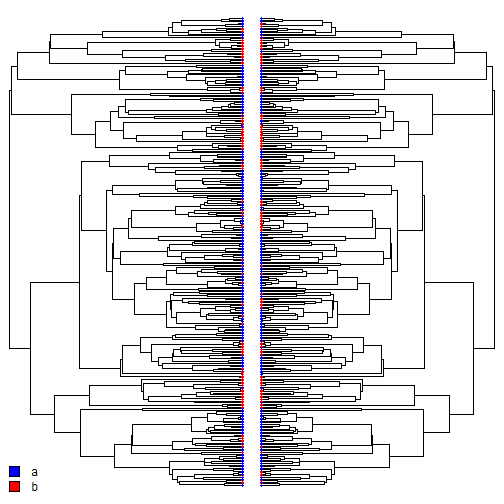``````par(fg="black")
``````

There may be a pattern of association, but from a visual inspection it's hard to tell.

OK, so we can repeat that also with the third column of `X`:

``````par(mfrow=c(1,2))
plot(tree,show.tip.label=FALSE,no.margin=TRUE)
par(fg="transparent")
tiplabels(pie=to.matrix(x[tree\$tip.label],c("a","b")),piecol=c("blue","red"),
cex=0.3)
par(fg="black")
add.simmap.legend(colors=setNames(c("blue","red"),c("a","b")),prompt=FALSE,
x=0,y=10,fsize=1)
par(fg="transparent")
plot(tree,show.tip.label=FALSE,no.margin=TRUE,direction="leftwards")
tiplabels(pie=to.matrix(z[tree\$tip.label],c("a","b")),piecol=c("blue","red"),
cex=0.3)
``````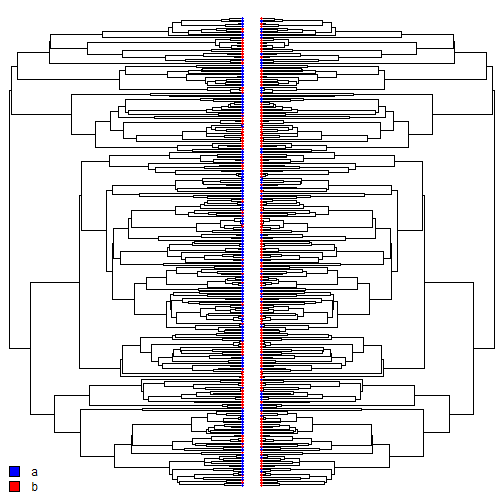``````par(fg="black")
``````

Once again, it's hard to tell whether or not the two characters have evolved in a coordinated fashion.

Now we're ready to run our models. First we will fit a model in which `x` depends on `y`, and vice versa; then we will fit a model in which `x` depends on `z` and the reverse.

``````fit.xy<-fitPagel(tree,x,y)
fit.xy
``````
``````##
## Pagel's binary character correlation test:
##
## Assumes "ARD" substitution model for both characters
##
## Independent model rate matrix:
##            a|a        a|b        b|a        b|b
## a|a -1.6338771  0.7929163  0.8409608  0.0000000
## a|b  0.4595895 -1.3005503  0.0000000  0.8409608
## b|a  0.5940171  0.0000000 -1.3869334  0.7929163
## b|b  0.0000000  0.5940171  0.4595895 -1.0536067
##
## Dependent (x & y) model rate matrix:
##            a|a        a|b        b|a        b|b
## a|a -1.0668077  0.6538038  0.4130039  0.0000000
## a|b  0.9094226 -4.6819126  0.0000000  3.7724900
## b|a  2.6064834  0.0000000 -4.2118641  1.6053807
## b|b  0.0000000  0.3624440  0.3440542 -0.7064982
##
## Model fit:
##             log-likelihood      AIC
## independent      -233.0357 474.0715
## dependent        -206.5951 429.1903
##
## Hypothesis test result:
##   likelihood-ratio:  52.88121
##   p-value:  9.023704e-11
##
## Model fitting method used was fitMk
``````
``````fit.xz<-fitPagel(tree,x,z)
fit.xz
``````
``````##
## Pagel's binary character correlation test:
##
## Assumes "ARD" substitution model for both characters
##
## Independent model rate matrix:
##            a|a        a|b        b|a        b|b
## a|a -2.1937429  1.3527818  0.8409611  0.0000000
## a|b  0.8686789 -1.7096399  0.0000000  0.8409611
## b|a  0.5940193  0.0000000 -1.9468011  1.3527818
## b|b  0.0000000  0.5940193  0.8686789 -1.4626982
##
## Dependent (x & y) model rate matrix:
##            a|a        a|b        b|a        b|b
## a|a -2.1476611  1.7078884  0.4397727  0.0000000
## a|b  0.9879923 -1.9819688  0.0000000  0.9939766
## b|a  0.3351617  0.0000000 -1.0123439  0.6771822
## b|b  0.0000000  0.6617982  0.6723597 -1.3341580
##
## Model fit:
##             log-likelihood      AIC
## independent      -248.4293 504.8585
## dependent        -246.8280 509.6559
##
## Hypothesis test result:
##   likelihood-ratio:  3.202592
##   p-value:  0.5245125
##
## Model fitting method used was fitMk
``````

Our results suggest that, indeed, `x` and `y` (but not `x` and `z`) may be co-evolving. Let's use the S3 `plot` method to visualize the result:

``````plot(fit.xy)
``````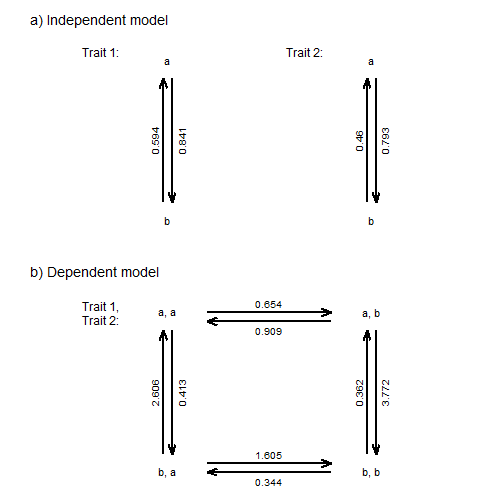``````## or
plot(fit.xy,lwd.by.rate=TRUE)
``````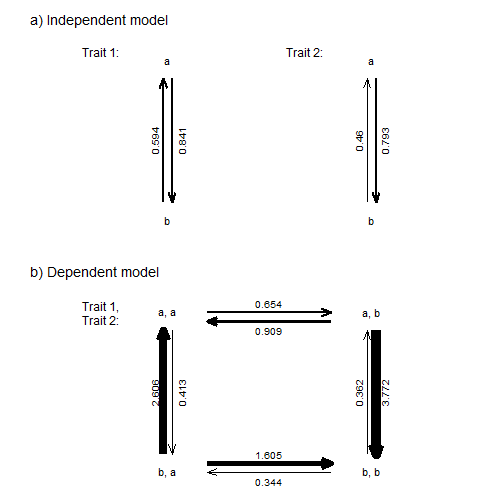We can also fit a model in which x depends on y, but not the converse, or y depends on x, but not the converse. We'll focus on `x` & `y` only because `x` and `z` do not appear to be correlated at all.

To do this we can run the following:

``````fit.x<-fitPagel(tree,x,y,dep.var="x")
fit.x
``````
``````##
## Pagel's binary character correlation test:
##
## Assumes "ARD" substitution model for both characters
##
## Independent model rate matrix:
##            a|a        a|b        b|a        b|b
## a|a -1.6338771  0.7929163  0.8409608  0.0000000
## a|b  0.4595895 -1.3005503  0.0000000  0.8409608
## b|a  0.5940171  0.0000000 -1.3869334  0.7929163
## b|b  0.0000000  0.5940171  0.4595895 -1.0536067
##
## Dependent (x only) model rate matrix:
##            a|a        a|b        b|a        b|b
## a|a -1.1647923  0.7981948  0.3665975  0.0000000
## a|b  0.4506321 -5.1481654  0.0000000  4.6975333
## b|a  3.0144648  0.0000000 -3.8126596  0.7981948
## b|b  0.0000000  0.3205196  0.4506321 -0.7711517
##
## Model fit:
##             log-likelihood      AIC
## independent      -233.0357 474.0715
## dependent        -207.6239 427.2478
##
## Hypothesis test result:
##   likelihood-ratio:  50.8237
##   p-value:  9.19968e-12
##
## Model fitting method used was fitMk
``````
``````plot(fit.x,lwd.by.rate=TRUE)
``````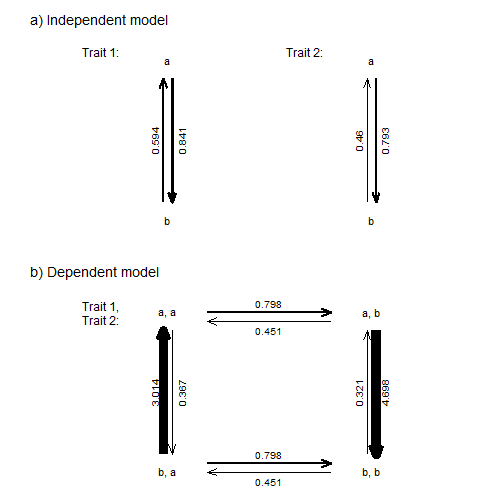``````fit.y<-fitPagel(tree,x,y,dep.var="y")
fit.y
``````
``````##
## Pagel's binary character correlation test:
##
## Assumes "ARD" substitution model for both characters
##
## Independent model rate matrix:
##            a|a        a|b        b|a        b|b
## a|a -1.6338771  0.7929163  0.8409608  0.0000000
## a|b  0.4595895 -1.3005503  0.0000000  0.8409608
## b|a  0.5940171  0.0000000 -1.3869334  0.7929163
## b|b  0.0000000  0.5940171  0.4595895 -1.0536067
##
## Dependent (y only) model rate matrix:
##            a|a        a|b        b|a        b|b
## a|a -1.2400483  0.3923515  0.8476968  0.0000000
## a|b  3.1664553 -4.0141521  0.0000000  0.8476968
## b|a  0.6916106  0.0000000 -4.6332640  3.9416534
## b|b  0.0000000  0.6916106  0.1515677 -0.8431783
##
## Model fit:
##             log-likelihood      AIC
## independent      -233.0357 474.0715
## dependent        -213.2927 438.5854
##
## Hypothesis test result:
##   likelihood-ratio:  39.48603
##   p-value:  2.665125e-09
##
## Model fitting method used was fitMk
``````
``````plot(fit.y,lwd.by.rate=TRUE)
``````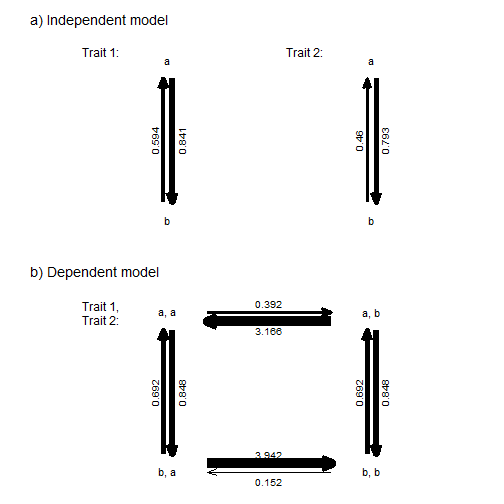Now we have fit four different models for the (co)evolution of `x` and `y`. One in which they evolve independently; one in which `x` depends on `y`, but not the reverse; one in which `y` depends on `x`, but not the reverse; and, finally, one in which `x` and `y` are interdependent. We can compare these different possibilities using AIC:

``````aic<-setNames(c(fit.xy\$independent.AIC,
fit.x\$dependent.AIC,
fit.y\$dependent.AIC,
fit.xy\$dependent.AIC),
c("independent","dependent x",
"dependent y","dependent x&y"))
aic
``````
``````##   independent   dependent x   dependent y dependent x&y
##      474.0715      427.2478      438.5854      429.1903
``````
``````aic.w(aic)
``````
``````##   independent   dependent x   dependent y dependent x&y
##    0.00000000    0.72355637    0.00249763    0.27394600
``````

This shows that the strongest weight of evidence supports the dependent x model, although interdependent x & y is also reasonably strongly supported.

Written by Liam J. Revell. Last updated 28 July 2018.